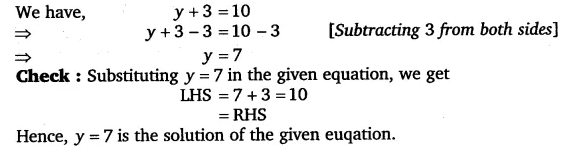# Solve the following equations:y + 3 = 10

Solve the following equation:
y + 3 = 10